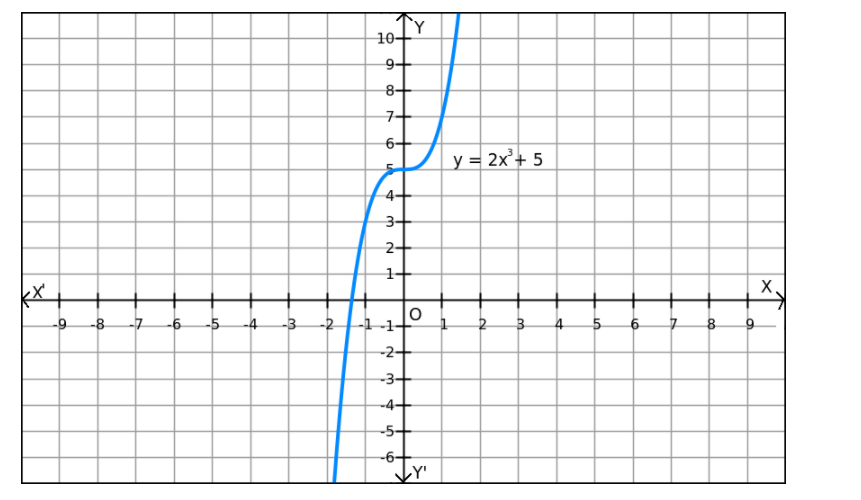# If the solve the problem`
Question:

$f(x)=2 x^{3}+5$ on $R$

Solution:

We can observe that f(x) increases when the values of x are increased and f(x) decreases when the values of x are decreased. Also, f(x) can be reduced by giving small values of x.
Similarly, f(x) can be enlarged by giving large values of x.
So, f(x) does not have a minimum or maximum value.